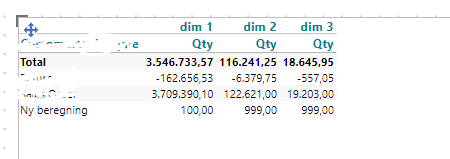# Add Row Numbers or use Absolute Reference in an IF calculation

(Targit Version 2019.4 build 17106)

I have a column where I need to use a different calculation depending on the row.

I know row 3 and 5 will always be the rows needing a different calculation so thought I'd just use a row number to reference in my calculation i.e. If columnrow 3 and 5 do calculations1 else calculations2 etc. but I can't seem to get a row number on all rows.

I tried allcount(0, all, m1) and it only works against Dimension rows and does not include calculated rows, I don't get an option to 'calculate in intersection', any ideas?

Other option would be to reference the User Dimension name direct but is this possible within an IF statement i.e. something like: If (absolute references sum(0,@"[Returns %]",0,0) then calculations1 else calculations2

All ideas and help much appreciated

• Hi Jason,

• Hi Marlene,

Sure thing, the green boxes I want to apply a different calculation.

Demand, Returns and Sales are User Dimensions and Returns %, Gross Margin and GM% are calculated rows.

(I'll add format not my choosing, it's finance wanting exact format they have in current Excel so I can't change layout unfortunately)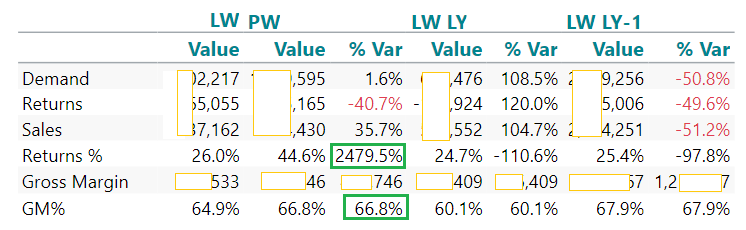• Hi Jason,

Maybe something like:

Just change the measure reference if wrong, but it seems from your picture that m3 is the ones you want to alter.

`if allcount(0,d1:0,m3) = 3 then "the formula for when it is row 3" else if allcount(0,d1:0,m3) = 5 "the formula for when it is row 5"Else "the normal formula"  `

• Thanks Kristoffer for the suggestion :-)

Yeah I tried that previously and ran into the problem of it only works against Dimension rows and does not include calculated rows, for instances.

This doesn't look at Calculated Rows and no at intersection option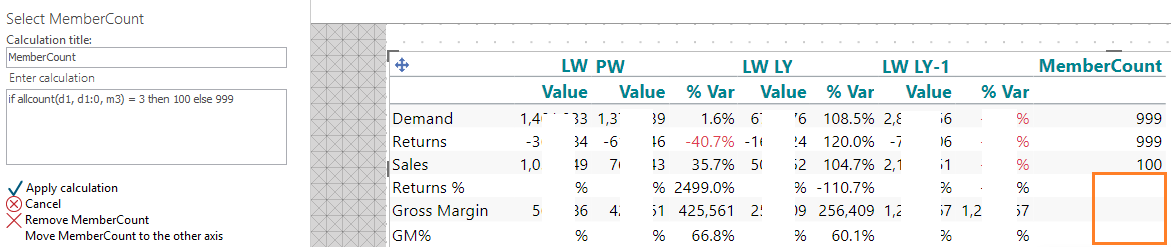If I do a basic calculation I can see the 'in intersection'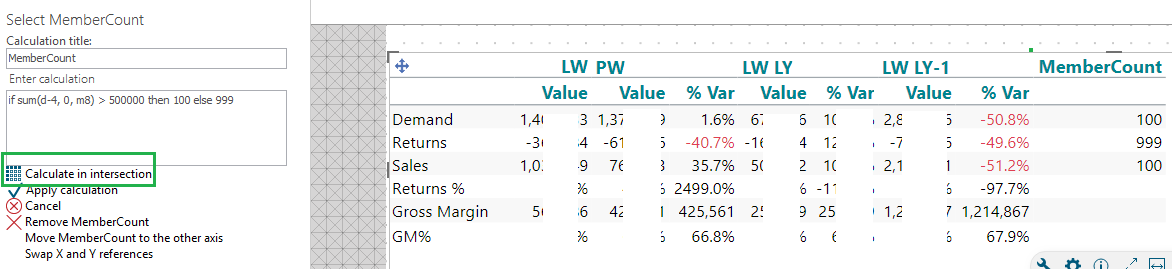and then it applies to all rows.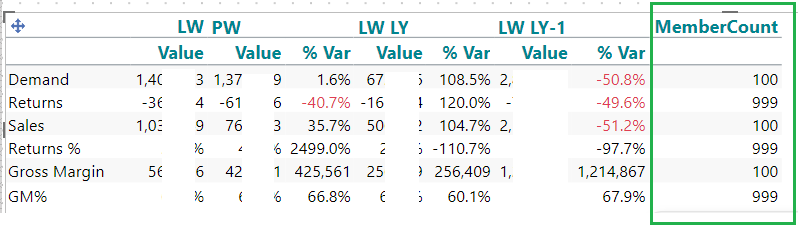Ooo these finance characters wanting everything to look like their Excel independent cells. Think I may have to switch this format up and split the crosstab and get them looking at the data in a different way.

• Hi Jason

Try this then, create a new measure, just for counting so you just set it to 1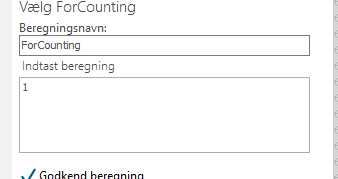In my example the count measure is m2

Therefor for row 3 I do this:

`if sum(0, all, m2) + 1 = 3 then 100 else 999`

And for row 5 this:

`if sum(0, all, m2) + 3 = 5 then 125 else 999`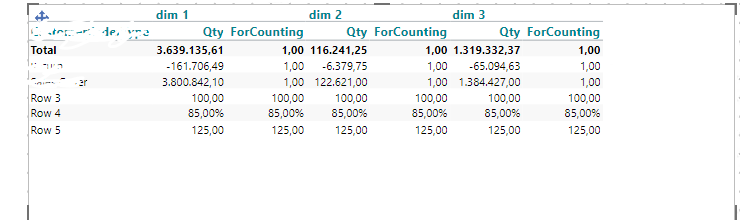And then you just hide the ForCounting column and you're left with: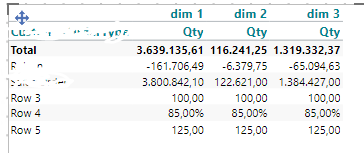• Thanks Kristoffer I will give it a go but I don't think it will work as we would now be removing the original calculation from row3 for the other columns, I'll let you know once tested.

Fingers crossed, Jason

• Hi Jason,

So it should only be row 3, and then the first column dimension?

In That case you can make a second counting column:

`allcount(d1:0, 0, m2)`

And then for the rows this:

In this case row 3

`if sum(0, all, m2) + 1 = 3 and sum(0, d1, m3) = 1 then 100 else 999`

And again hide both columns, and you're left with